# Search

About 547 Search Results Matching Types of Worksheet, Worksheet Section, Generator, Generator Section, Grades matching 3rd Grade, Similar to Easy Measurement Worksheets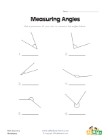## Measuring Angles Worksheet 2

Use a protractor to measure each of the angles.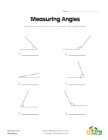## Measuring Angles Worksheet 1

Measure each of the angles with a protractor an...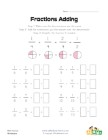## Easy Fraction Addition with Reducing Worksheet

Simple fraction addition problems with reducing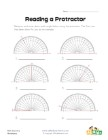## Reading a Protractor Worksheet 2

Read each protractor and write down the angles ...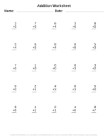Generate printable addition worksheets using ou...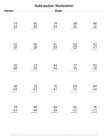## Subtraction Worksheet Generator

Generate printable subtraction worksheets using...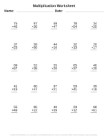## Multiplication Worksheet Generator

Generate printable multiplication worksheets us...